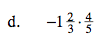### Home > MC2 > Chapter 6 > Lesson 6.1.3 > Problem6-30

6-30.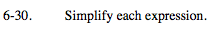Find a common denominator and simplify.

$\left(-\frac{4}{5}\right)\left(\frac{12}{12}\right) + \left(\frac{7}{12}\right)\left(\frac{5}{5}\right)$

$-\frac{13}{60}$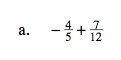Remember that adding a negative number is essentially subtraction.

$\frac{11}{36}$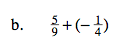Multiply the numerators together and the denominators together.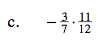Turning the first fraction into an improper fraction may prove to be more helpful.